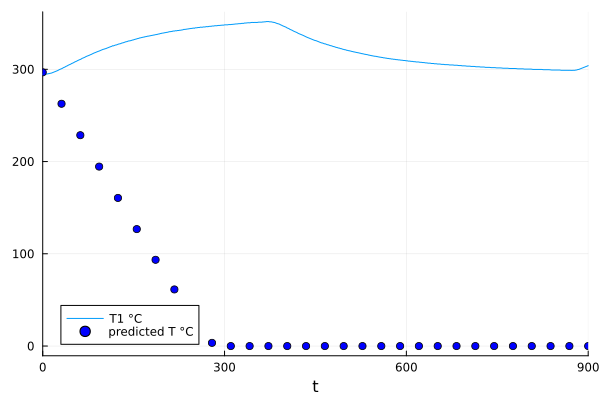# Running neural ODE on measurement

I am trying get a neural ODE working, that doesn’t have a function/ode as input but a measurement.
I want to apply the neural ODE to get the original function expressed by the measurement and not the derivative.

I am trying to use this three websites:

But the sites use different librarys Lux and Flux also do not contain a simple example of how input the measurement.

For measurement I again use:
heating Q in percent 0 to 100 in X
and resulting temperature in Kelvin. The starting temperature is: 296.693 K.

This the code I came up with so far

``````u0 = Float64[296.693, ]
tspan = (0.0, 8_000)
tsteps = range(tspan, tspan, length=30)

dudt = Flux.Chain(Flux.Dense(1=>32, tanh),
Flux.Dense(32=>1)) |> f64
u = static_df.Q1
y = static_df.T1

n_ode = NeuralODE(dudt, tspan, Tsit5(), saveat=tsteps, reltol=1e-7,abstol=1e-9)
ps = Flux.params(n_ode)

function predict_n_ode(u)
n_ode(u)
end

loss_n_ode() = sum(abs2,y .- predict_n_ode(u))

Flux.train!(loss_n_ode, ps, (u, y), opt_n_ode)
``````

I get the error: `no method matching loss_n_ode(::Vector{Float64})`. Which makes perfectly sense, I just don’t know. How I should have done it instead.

What is your model? Can you write down what you’re trying to do at a higher level in just the math first?

I have taken a measurement of cooling and heating curves.

Models are for example:

or:

What I am trying to reach is to be able to predict the temperature curve under the condition heating is on/off.

I want to feed in the heating percentages [0, 100] and recreate the measured temperatures. The neural network shall learn the function heating → temperature.

Define `u` as a continuous function from data using

and then call it from within the ODE definition.

Okay,

I tried different things, including the interpolation.

My current latest try is:

``````	u0 = Float64[296.693, ]
tspan = (0.0, 8_000)
tsteps = range(tspan, tspan, length=30)

dudt = Flux.Chain(Flux.Dense(1=>32, tanh),
Flux.Dense(32=>1)) |> f64
u = static_df.Q1
y = static_df.T1
interp = LinearInterpolation(u,y)

n_ode = NeuralODE(dudt, tspan, Tsit5(), saveat=tsteps, reltol=1e-7,abstol=1e-9)
ps = Flux.params(n_ode)

function predict_n_ode(u)
n_ode([u, ])
end

loss_n_ode(x) = sum(abs2,y .- predict_n_ode(x))

Flux.train!(loss_n_ode, ps, u, opt_n_ode)
``````

The pluto cell is currently running two minutes and has a progress bar of 1 %. So I think, something is runninng. But it has no interpolation and I still don’t know, why to write the predict_n_node function this way.

This sounds like a linear system, are you sure you need neural networks for this task? Here’s an example estimating a simple model for a heating system
https://baggepinnen.github.io/ControlSystemIdentification.jl/dev/examples/temp/

1 Like

Okay although the code did run. It was completely wrong. The result was a probably the stacking 1D input data.
In Addition it did not optimize.
@baggepinnen You are right. An neural ODE should be a complete overdo for a heating system.
The aim is more to have a real world toy example.
@ChrisRackauckas hinted to use a interpolation. I tried this again:

``````
u0 = Float64[296.693, ]

end_point = 900
tspan = (0.0, end_point)
tsteps = range(tspan, tspan, length=30)

dudt = Flux.Chain(Flux.Dense(1=>32, tanh),
Flux.Dense(32=>1)) |> f64

t = static_df[1:end_point, :time] .- static_df.start_time
u = static_df[1:end_point, :Q1]
u_t = LinearInterpolation(u, t)
y = static_df[1:end_point, :T1]
y_t = LinearInterpolation(y, t)
ode_data = Array(y_t(tsteps))

n_ode = NeuralODE(dudt, tspan, Tsit5(), saveat=tsteps, reltol=1e-7,abstol=1e-9)
``````

I was able to use u0, to predict before the training.Than I tried hard to adept the loss function and still follow the example as close as I can.

``````ps = Flux.params(n_ode)

function predict_n_ode()
n_ode(u0)
end

loss_n_ode() = sum(abs2,ode_data .- transpose(predict_n_ode()))

You didn’t use `u` at all in your loss function? Define your ODE so it has the NN with the interpolation in there instead of using the NeuralODE primitive.